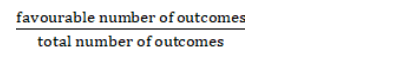# From a well-shuffled pack of 52 cards, a card is drawn at random.`
Question:

From a well-shuffled pack of 52 cards, a card is drawn at random. Find the probability of its being a king or a queen

Solution:

let A denote the event that the card drawn is king and B denote the event that card drawn is queen.

In a pack of 52 cards, there are 4 king cards and 4 queen cards

Given : $P(A)=\frac{4}{52}, P(B)=\frac{4}{52}$

To find : Probability that card drawn is king or queen $=P(A$ or $B)$

The formula used : Probability =$P(A$ or $B)=P(A)+P(B)-P(A$ and $B)$

$P(A)=\frac{4}{52}$ (as favourable number of outcomes $=4$ and total number of outcomes $=52$ )

$P(B)=\frac{4}{52}$ (as favourable number of outcomes $=4$ and total number of outcomes $\left.=52\right)$

Probability that card drawn is king or queen = P(A and B)= 0

(as a card cannot be both king and queen in the same time)

$P(A$ or $B)=\frac{4}{52}+\frac{4}{52}-0$

$P(A$ or $B)=\frac{4+4}{52}=\frac{8}{52}=\frac{2}{13}$

$P(A$ or $B)=\frac{2}{13}$

Probability of a card drawn is king or queen $=P(A$ or $B)=\frac{2}{13}$